## Suppose that two sources of sound produce waves of the same exact amplitude and frequency, but are out of phase by one-half of a wavelength.

Question

Suppose that two sources of sound produce waves of the same exact amplitude and frequency, but are out of phase by one-half of a wavelength. What will the end result be?

A. You would hear two different sounds of equal volume and pitch.

B. You would hear a sound that’s twice as intense, since the waves are complementing each other.

C. You would hear pulsations in the waves at certain intervals, known as beats.

D.You wouldn’t hear any sound since the waves are canceling each other out.

in progress 0
2 months 2021-07-30T21:02:54+00:00 2 Answers 4 views 0

D) You wouldn’t hear any sound since the waves are canceling each other out.

Explanation:

Suppose two sound waves with same amplitude,frequency and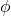phase difference are given by as follows,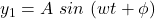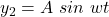Since waves are out of phase by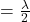, phase difference=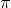hence, resultant displacement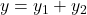=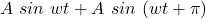=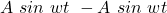=0

Hence we wouldn’t hear any sound since the waves are canceling each other out.